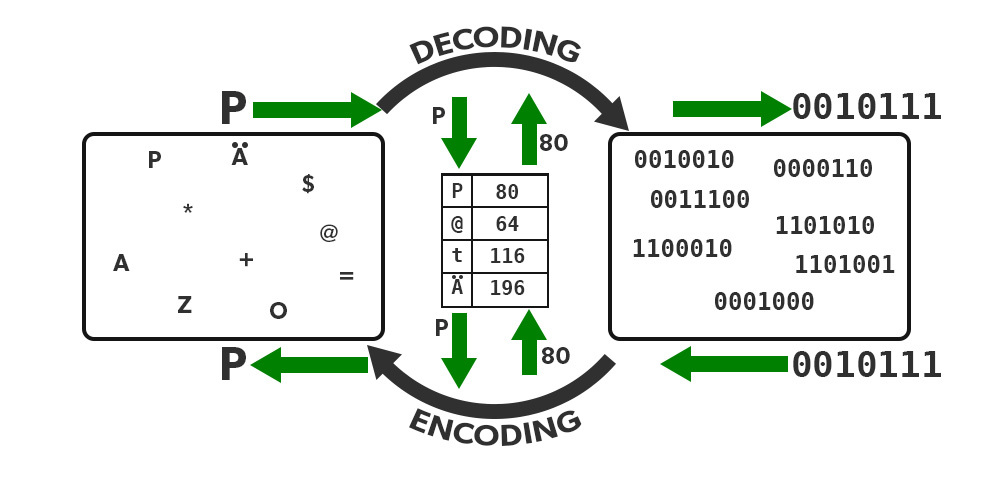Open In App

# Java Program to Store Unicode Characters Using Character Literals

ASCII table helps to get computation done earlier but with the ongoing passage of time, there emerge several human-readable languages where the constraint with ASCII was it only supports 256 symbols that too of English only. Now to make computations for other languages there emerges a UNICODE system for computation inside the computer that supports 65535 symbols.

Unicode characters are universal character encoding standards. It represents the way different characters can be represented in different documents like text files, web pages, etc. The Unicode supports 4 bytes for the characters. UTF-8 has become standard character encoding it supports 4 bytes for each character. There are other different Unicode encodings like UTF-16 and UTF-8. Character literals in Java are constant characters in Java. They are expressed in single quotes ‘a’,’A, ‘1’,’!’, ‘π’, ‘\$’,’©’. The data type that can store char literals is char.Different Methods to Store Unicode Characters

1. Assigning Unicode to the char data types
2. Assigning Unicode values to char data types
3. Assigning ASCII values to char data types

Now let us discuss the above methods by listing the approach and lately implementing the same that is as follows:

Method 1: Assigning Unicode to the char data types.

Illustrations:

```Input : a = '\$'
Output: \$```
```Input : a = '~'
Output: ~```

Approach:

1. Create a character variable.
2. Store Unicode characters in a variable using a single quote.
3. Print the variable on the console.

Example:

## Java

 `// Java Program to Store Unicode Characters``// Using Character Literals``// By Assigning Unicode to the char data types` `// Importing required classes``import` `java.io.*;` `// Class``class` `GFG {` `    ``// Main driver method``    ``public` `static` `void` `main(String[] args)``    ``{` `        ``// Declaring and initializing character``        ``// lately printing the same on console``        ``char` `c1 = ``'a'``;``        ``System.out.println(c1);` `        ``char` `c2 = ``'A'``;``        ``System.out.println(c2);` `        ``char` `c3 = ``'1'``;``        ``System.out.println(c3);` `        ``char` `c4 = ``'~'``;``        ``System.out.println(c4);` `        ``char` `c5 = ``'\$'``;``        ``System.out.println(c5);` `        ``char` `c6 = ``'/'``;``        ``System.out.println(c6);` `        ``char` `c7 = ``'π'``;``        ``System.out.println(c7);``    ``}``}`

Output

```a
A
1
~
\$
/
?```

Method 2: Assigning Unicode values to char data types

Illustrations:

```Input  : a = '\u0061'
Output : a```
```Input  : a = '\u002F'
Output : /```

Approach:

1. Create a char variable.
2. Store Unicode value in a variable using a single quote.
3. Print the variable on the console.

Example

## Java

 `// Java Program to Store Unicode Characters``// Using Character Literals``// By Assigning Unicode Values to char Data Types` `// Importing required classes``import` `java.io.*;` `// Class``class` `GFG {` `    ``// Main driver method``    ``public` `static` `void` `main(String[] args)``    ``{` `        ``// Declaring and initializing character``        ``// lately printing the same on console``        ``char` `c1 = ``'\u0061'``;``        ``System.out.println(c1);` `        ``char` `c2 = ``'\u0041'``;``        ``System.out.println(c2);` `        ``char` `c3 = ``'\u0031'``;``        ``System.out.println(c3);` `        ``char` `c4 = ``'\u007E'``;``        ``System.out.println(c4);` `        ``char` `c5 = ``'\u0024'``;``        ``System.out.println(c5);` `        ``char` `c6 = ``'\u002F'``;``        ``System.out.println(c6);` `        ``char` `c7 = ``'\u03C0'``;``        ``System.out.println(c7);``    ``}``}`

Output

```a
A
1
~
\$
/
?```

Method 3: Assigning ASCII values to char data types

Illustrations:

```Input : a = 97
Output: a```
```Input : a = 49
Output: 1```

Approach:

1. Create a character variable.
2. Store ASCII value in a variable using a single quote.
3. Print the variable on the console.

Example:

## Java

 `// Java Program to Store Unicode Characters``// Using Character Literals``// By Assigning ASCII Values``// to char Data Types` `// Importing required classes``import` `java.io.*;` `// Class``class` `GFG {` `    ``// Main driver method``    ``public` `static` `void` `main(String[] args)``    ``{` `        ``// Declaring and initializing character``        ``// lately printing the same on console``        ``char` `c1 = ``97``;``        ``System.out.println(c1);` `        ``char` `c2 = ``65``;``        ``System.out.println(c2);` `        ``char` `c3 = ``49``;``        ``System.out.println(c3);` `        ``char` `c4 = ``126``;``        ``System.out.println(c4);` `        ``char` `c5 = ``36``;``        ``System.out.println(c5);` `        ``char` `c6 = ``47``;``        ``System.out.println(c6);``    ``}``}`

Output

```a
A
1
~
\$
/```